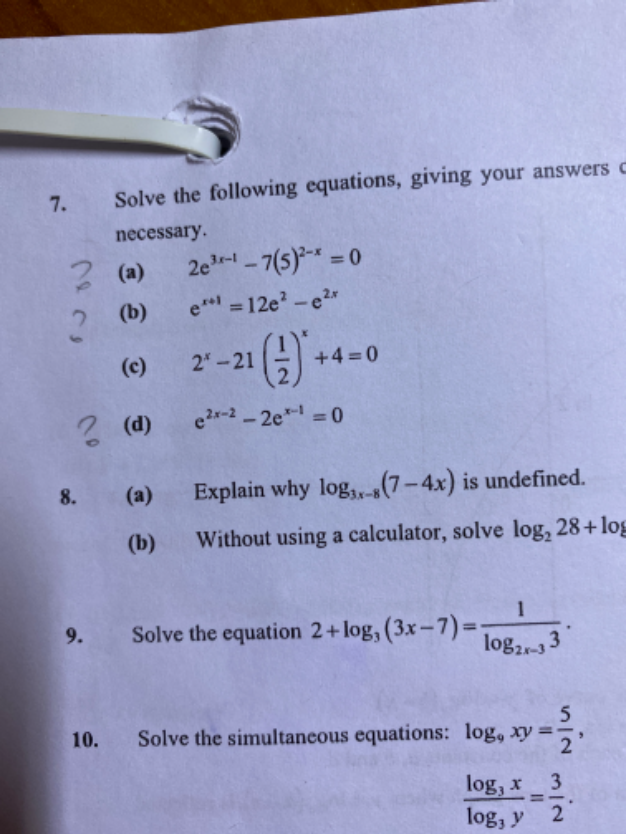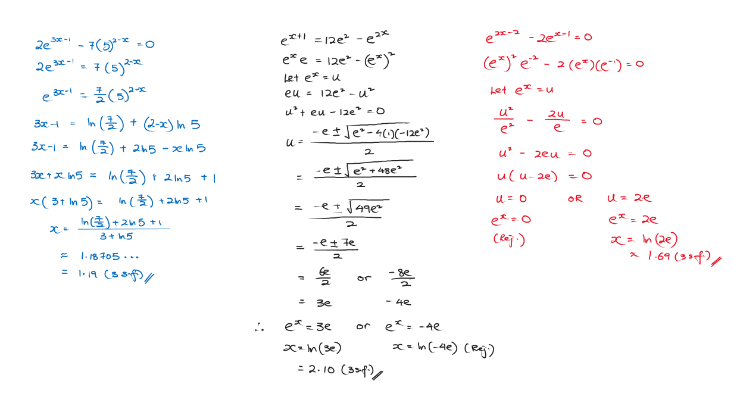# QuestionKindly solve a b and d thanks

(a) is the same as Adwin’s solution

(b) and (d) , I have alternative approach but answers are the same.

Alternative solution for (b), I used completing the square method.

ex+1  = 12e – e2x

ex . e  = 12e – (ex )2

let A = ex

A. e = 12e – A2

A2 +  A. e   =  12e2

[A2 +  A. e  +  (e/2)2 ] –  (e/2)2  =  12e2

[ A – (e/2) ]2  = 12e2 +  (e/2)2

[ A – (e/2) ] = (48/4)e2 + e2 /4 = (49/4) e2

A – (e/2)  =  ±   [(49/4) e2 ] = ± (7/2)e

A = (7/2)e –  (e/2)  = 3e       or    A  =  – (7/2)e –  (e/2) = -4e

A = e cannot be negative so A = -4e   is extraneous

Hence   ex  = 3e  => ln ex = ln 3  =>  x ~ 2.10  (to 3 s.f)

Alternative solution for (c)

e2x-2 – 2ex-1  = 0

let A = ex-1

A2 -2A = 0

A(A-2) = 0  => A = 0 or A = 2  but A= 0 is extraneous

Hence  ex-1  = 2

ln ex-1  = ln 2

x – 1 = ln 2

x = ln 2 + 1    ~ 1.69 ( to 3 s.f)

1 Reply 1 Like

Thanks Sushi 88.

1 Reply 0 Likes1 Reply 1 Like